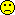## We were all blind (except Detlew) [🇷 for BE/BA]

Hi ElMaestro,

we were all blind!
Just to make clear what I mean by “we”: Myself, you, the EMA’s PKWP (and the BSWP fabricating the example datasets and the evaluation by ‘Method C’), and Patterson & Jones.
Detlew read the guidance’s Step 1 thoroughly enough to realize that $$\small{s_\textrm{wR}^2}$$ is not an REML-estimate (see this post). Fortunately the templates for Phoenix are correct. Quick & dirty R-code at the end. Results:

      file             descr       s2wR       swR CVwR (%)      PHX match   ds02.csv            EMA II 0.01298984 0.1139730 11.43441 11.43441   yes  ds02a.csv     EMA II imbal. 0.01038611 0.1019123 10.21774 10.21774   yes   DS04.csv Patterson & Jones 0.32489813 0.5699984 61.95883 61.95883   yes   ds01.csv             EMA I 0.2188968  0.4678640 46.96431 46.96431   yes

In the Q&A we find for DSII the REML-based CVwR 11.5% (PHX 11.43%). Patterson & Jones reported for their dataset 61% (SAS); I got in PHX 61.96%. For DSI the Q&A gives 47.3% (PHX 46.96%).

Therefore, congratulations to all of us answering a question which was not asked by the FDA.GetData <- function(path) {   D        <- NULL # paranoia   D        <- read.csv(path, comment.char = "#")   D        <- D[, 1:5]   D[, 5]   <- log(D[, 5])   names(D) <- c("Subj", "Per", "Seq", "Trt", "PK")   return(invisible(D)) } CalcSwR <- function(path) {   D    <- GetData(path)   R    <- D[D$Trt == "R", ] R <- cbind(R, Diff = NA) seqs <- sort(unique(D$Seq), decreasing = TRUE)   des  <- NA   if (sum(seqs %in% c("TRR", "RTR", "RRT")) == 3) des <- "partial"   if (sum(seqs %in% c("TRTR", "RTRT")) == 2) des <- "full"   if (is.na(des)) stop("Design not supported.")   if (des == "partial") {     TRR <- R[R$Seq == "TRR", c(1:3, 5:6)] RTR <- R[R$Seq == "RTR", c(1:3, 5:6)]     RRT <- R[R$Seq == "RRT", c(1:3, 5:6)] # keep only subjects with two observations TRR <- TRR[duplicated(TRR$Subj, fromLast = TRUE) |                duplicated(TRR$Subj, fromLast = FALSE), ] RTR <- RTR[duplicated(RTR$Subj, fromLast = TRUE) |                duplicated(RTR$Subj, fromLast = FALSE), ] RRT <- RRT[duplicated(RRT$Subj, fromLast = TRUE) |                duplicated(RRT$Subj, fromLast = FALSE), ] # first minus second administration # too lazy to vectorize for (j in 1:(nrow(TRR)-1)) { if (TRR$Per[j] == 2) {         TRR$Diff[j] <- TRR$PK[j] - TRR$PK[j+1] } } for (j in 1:(nrow(RTR)-1)) { if (RTR$Per[j] == 1) {         RTR$Diff[j] <- RTR$PK[j] - RTR$PK[j+1] } } for (j in 1:(nrow(RRT)-1)) { if (RRT$Per[j] == 1) {         RRT$Diff[j] <- RRT$PK[j] - RRT$PK[j+1] } } R <- rbind(TRR[, c(3, 5)], RTR[, c(3, 5)], RRT[, c(3, 5)]) } else { TRTR <- R[R$Seq == "TRTR", c(1:3, 5:6)]     RTRT <- R[R$Seq == "RTRT", c(1:3, 5:6)] TRTR <- TRTR[duplicated(TRTR$Subj, fromLast = TRUE) |                  duplicated(TRTR$Subj, fromLast = FALSE), ] RTRT <- RTRT[duplicated(RTRT$Subj, fromLast = TRUE) |                  duplicated(RTRT$Subj, fromLast = FALSE), ] for (j in 1:(nrow(TRTR)-1)) { if (TRTR$Per[j] == 2) {         TRTR$Diff[j] <- TRTR$PK[j] - TRTR$PK[j+1] } } for (j in 1:(nrow(RTRT)-1)) { if (RTRT$Per[j] == 1) {         RTRT$Diff[j] <- RTRT$PK[j] - RTRT$PK[j+1] } } R <- rbind(TRTR[, c(3, 5)], RTRT[, c(3, 5)]) } R <- R[!is.na(R$Diff), ]   m    <- lm(Diff ~ as.factor(Seq), data = R)   s2wR <- anova(m)["Residuals", "Mean Sq"]/2   res  <- c(s2wR = s2wR, swR = sqrt(s2wR), CVwR = 100*sqrt(exp(s2wR)-1))   names(res) <- "CVwR (%)"   return(res) } p <- paste0("https://",        c("bebac.at/downloads/ds02.csv",          "bebac.at/downloads/ds02a.csv",          "raw.githubusercontent.com/Helmut01/replicateBE/master/inst/extdata/DS04.csv",          "bebac.at/downloads/ds01.csv")) d <- c("EMA II", "EMA II imbal.", "Patterson & Jones", "EMA I") res <- data.frame(file = basename(p), descr = d, X1 = NA, X2 = NA, X3 = NA,                   PHX = c(11.43441, 10.21774, 61.95883, 46.96431), match = "no") names(res)[3:5] <- c("s2wR", "swR", "CVwR (%)") for (j in 1:4) {   res[j, 3:5] <- CalcSwR(path = p[j])   if (round(res[j, 5], 5) == res$PHX[j]) res$match[j] <- "yes" } print(res, row.names = FALSE)

Dif-tor heh smusma 🖖Helmut SchützThe quality of responses received is directly proportional to the quality of the question asked. 🚮
Science QuotesIng. Helmut Schütz